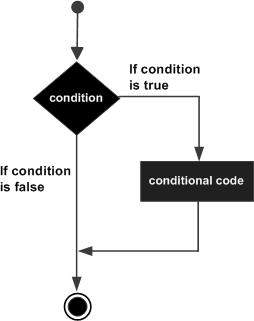# Python 3 - IF Statement

The IF statement is similar to that of other languages. The if statement contains a logical expression using which the data is compared and a decision is made based on the result of the comparison.

## Syntax

```if expression:
statement(s)
```

If the boolean expression evaluates to TRUE, then the block of statement(s) inside the if statement is executed. In Python, statements in a block are uniformly indented after the : symbol. If boolean expression evaluates to FALSE, then the first set of code after the end of block is executed.

## Flow Diagram## Example

```#!/usr/bin/python3

var1 = 100
if var1:
print ("1 - Got a true expression value")
print (var1)

var2 = 0
if var2:
print ("2 - Got a true expression value")
print (var2)
print ("Good bye!")
```

## Output

When the above code is executed, it produces the following result −

```1 - Got a true expression value
100
Good bye!
```
python_decision_making.htm